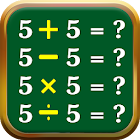Math Games - Maths Tricks

All Android applications categories

All Android games categories# Math Games - Maths Tricks

2K 8.6

8.6 Users
rating

## Screenshots

Description

Math Games - Maths Tricks for everyone from kids to adults. Mathematical calculations to play and practice with simple addition subtraction multiplication and division. The mathe game division calculator is division app for kids as the maths division app as the math division game also call math divide app as math divide game math division app as math divition at the matematica. This is best math game brain maths as excercise math free maths games very hard math games for kids math brain math game apps. The math game for adults as the addition games as the math test plusminus as simple mathematics apps.

Features of Math Games - Maths Tricks.

# Subtraction games subtracting numbers to solve the equations.
# Multiplication games multiplication tables learning and duel play mode.
# Division games practice and learn division tables.
# Maths times tables and hard games on maths.

Math Games - Maths Tricks in category has different play modes to improve math skills play learn quiz practice duel. Free math games can be educational learning for kids or brain training app for adults. This is maths solutions for any question and maths tricks is maths questions solving app as well as maths app as maths quiz. The maths games at the maths quiz games as the maths question solver app. The maths questions with answers as mathematics apps as mathematics books free at mathematics formula as well mathematics games mathematics games at the maths tables book free as the maths tables learn easy 1 to 30 as the maths tables games. The math games for adults offline as math master exercises. The mathematics addition math game as the multiplication and division games for 4th graders as the math practice set in hindi as the math practice set in hindi offline. In this app simple maths tricks in the simple maths for kids as the simple maths arithmetic tricks as the simple maths game arithmatic app or math apps math for kids. The simple addition for kids as the simple addition subtraction or simple multiplication or ganit sikhne ka tarika also ganit sikhne wala app at the ganit sikhne ke liye as math games for free subracting apps as simple mathematics games for kids all maths formula app as how to learn tables easily mathematics.

Math Games - Maths Tricks is maths practice game to train your brain & is designed for all ages including kids girls and boys adults including parents and grandparents. Smallest in size maths apps on google play easiest multiplication and division games with addition and subtraction games all in one app and maths tricks books free. The mathematics apps learn mathematics add subtract multiply & divide this is kides game and kides math as the math table math year 1. This is math year 1 as the math in hindi with solution at the brain games also mathmatics app in hindi at the mathmatics solution app for brain puzzle games for children as subtraction games for kids in this subtraction games 1st grade as the subtracting and adding at addition and subtraction games for kids as the hard math games for adults as hard mathematics game and mathematics hard games and math puzzle games as math practice app.

This addition subtraction multiplication and division games as addition subtraction and multiplication addition subtraction multiplication division. In this app addition subtraction app as the two digit addition and subtraction. This is easy math games for kids free easy maths learning app at the easy maths games easy maths quiz at the easy maths tricks. This is math answer app with solution multiplication table learning for kids at the multiplication table kids math as divides game.

Download and give us a review of Math Games - Maths Tricks.

## Users review

from 2.033 reviews

"Great"

8.6